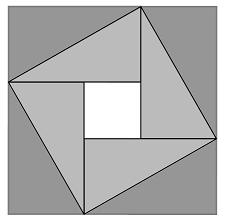You may also likeFitting In

The largest square which fits into a circle is ABCD and EFGH is a square with G and H on the line CD and E and F on the circumference of the circle. Show that AB = 5EF. Similarly the largest equilateral triangle which fits into a circle is LMN and PQR is an equilateral triangle with P and Q on the line LM and R on the circumference of the circle. Show that LM = 3PQPinned Squares

What is the total number of squares that can be made on a 5 by 5 geoboard?Zig Zag

Four identical right angled triangles are drawn on the sides of a square. Two face out, two face in. Why do the four vertices marked with dots lie on one line?

Just Opposite

Why do this problem?

This problem allows students to explore new ways to see (visualise) the 'tilted square' figure. It combines work on coordinates and area with possibel extensions into Pythagoras' theorem. There is the potential for different insights that can be utilised to invite discussion about different approaches.

Possible approach

A visualisation can be grasped quite easily when someone points it out, but it is more satisfying and much better for the students' development if they gradually feel their way around the structure with moments of revelation.

Invite learners to create tilted squares of their own, identify coordinates of diagonally opposite corners. Can they usethese to help to find areas? Share ideas and generalisations as they arise.

Connections may take time to emerge and different insights might result in different approaches. For example the area of the tilted square might be found through considering one of the squares and a rectangle or seen as half way between the areas of the smaller and larger squares. Give space for learners to find their own visualisation and share different ideas and approaches.

One important configuration to watch for is this one:Key questions

• Can you work form some specific cases to the general?
• How do the areas of the squares and rectangles relate?
• How do the coordinates of opposite coordinates relate to the dimensions of the inner and outer squares?

Possible extension

Students may be familiar already with a proof of Pythagoras' Theorem based on this. If not, this is a good moment to include it and connect the ideas associated with this form.
This may be a good next problem for abler students :Something in Common

Possible support

A problem which focuses on finding the areas of tilted squares is Making Squares .